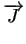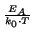Next: 2.1.1 Extrinsic Dopant Diffusion Up: 2. Physics of Diffusion Previous: 2. Physics of Diffusion

# 2.1 Diffusion

Diffusion is a mass transport phenomenon where in dependence of temperature molecular dynamic local exchanges take place. In case of unequal distributed concentrations the statistical average of molecular movements strives for balance the inequality. This molecular dynamic behavior leads to a macroscopic flux that can be described as a material transport caused by the gradient of concentrations known as Fick's first law.= - D . gradC(2.1)

where C denotes the concentration of the species,the diffusion flux of the particles and D the diffusion coefficient or also called diffusivity. D itself varies with temperature following the Arrhenius law
 D = D0 . e(2.2)

where D0 denotes a constant prefactor and EA is the activation energy.

The conservation of dopant atoms is expressed by a continuity equation and is known as Fick's second law= - div() (2.3)

In case of low doping concentrations (intrinsic diffusion) the measured diffusion profiles are well behaved and agree with Fick's laws. But at high doping concentrations the diffusion profiles deviate from the predictive calculations of Fick's laws [Sch91].Next: 2.1.1 Extrinsic Dopant Diffusion Up: 2. Physics of Diffusion Previous: 2. Physics of Diffusion